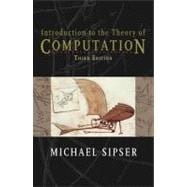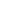# Introduction to the Theory of Computation

• ISBN13:

• ISBN10:

## 113318779X

• Edition: 3rd
• Format: Hardcover
• Copyright: 2012-06-27
• Publisher: Course Technology

Note: Supplemental materials are not guaranteed with Rental or Used book purchases.

Purchase Benefits

•Free Shipping On Orders Over \$35!
Your order must be \$35 or more to qualify for free economy shipping. Bulk sales, PO's, Marketplace items, eBooks and apparel do not qualify for this offer.
•Get Rewarded for Ordering Your Textbooks! Enroll Now
•Complimentary 7-Day eTextbook Access - Read more
When you rent or buy this book, you will receive complimentary 7-day online access to the eTextbook version from your PC, Mac, tablet, or smartphone. Feature not included on Marketplace Items.
List Price: \$271.99 Save up to \$243.59
• Rent Book \$36.58Free Shipping

TERM
PRICE
DUE7-Day eTextbook Access

IN STOCK USUALLY SHIPS IN 24 HOURS.

### Supplemental Materials

What is included with this book?

• The New copy of this book will include any supplemental materials advertised. Please check the title of the book to determine if it should include any access cards, study guides, lab manuals, CDs, etc.
• The Used, Rental and eBook copies of this book are not guaranteed to include any supplemental materials. Typically, only the book itself is included. This is true even if the title states it includes any access cards, study guides, lab manuals, CDs, etc.

### Summary

Gain a clear understanding of even the most complex, highly theoretical computational theory topics in the approachable presentation found only in the market-leading INTRODUCTION TO THE THEORY OF COMPUTATION, 3E. The number one choice for today's computational theory course, this revision continues the book's well-know, approachable style with timely revisions, additional practice, and more memorable examples in key areas. A new first-of-its-kind theoretical treatment of deterministic context-free languages is ideal for a better understanding of parsing and LR(k) grammars. You gain a solid understanding of the fundamental mathematical properties of computer hardware, software, and applications with a blend of practical and philosophical coverage and mathematical treatments, including advanced theorems and proofs. INTRODUCTION TO THE THEORY OF COMPUTATION, 3E's comprehensive coverage makes this a valuable reference for your continued studies in theoretical computing.

### Author Biography

Michael Sipser has taught theoretical computer science and other mathematical subjects at the Massachusetts Institute of Technology for the past 32 years. He is the Head of the Mathematics Department and a member of the Computer Science and Artificial Intelligence Laboratory (CSAIL). He enjoys teaching and pondering the many mysteries of complexity theory.

### Table of Contents

 Preface to the First Edition p. xi To the student p. xi To the educator p. xii The first edition p. xiii Feedback to the author p. xiii Acknowledgments p. xiv Preface to the Second Edition p. xvii Preface to the Third Edition p. xxi Introduction p. 1 Automata, Computability, and Complexity p. 1 Complexity theory p. 2 Computability theory p. 3 Automata theory p. 3 Mathematical Notions and Terminology p. 3 Sets p. 3 Sequences and tuples p. 6 Functions and relations p. 7 Graphs p. 10 Strings and languages p. 13 Boolean logic p. 14 Summary of mathematical terms p. 16 Definitions, Theorems, and Proofs p. 17 Finding proofs p. 17 Types of Proof p. 21 Proof by construction p. 21 Proof by contradiction p. 21 Proof by induction p. 22 Exercises, Problems, and Solutions p. 25 Automata and Languages p. 29 Regular Languages p. 31 Finite Automata p. 31 Formal definition of a finite automaton p. 35 Examples of finite automata p. 37 Formal definition of computation p. 40 Designing finite automata p. 41 The regular operations p. 44 Nondeterminism p. 47 Formal definition of a nondeterministic finite automaton p. 53 Equivalence of NFAs and DFAs p. 54 Closure under the regular operations p. 58 Regular Expressions p. 63 Formal definition of a regular expression p. 64 Equivalence with finite automata p. 66 Nonregular Languages p. 77 The pumping lemma for regular languages p. 77 Exercises, Problems, and Solutions p. 82 Context-Free Languages p. 101 Context-Free Grammars p. 102 Formal definition of a context-free grammar p. 104 Examples of context-free grammars p. 105 Designing context-free grammars p. 106 Ambiguity p. 107 Chomsky normal form p. 108 Pushdown Automata p. 111 Formal definition of a pushdown automaton p. 113 Examples of pushdown automata p. 114 Equivalence with context-free grammars p. 117 Non-Context-Free Languages p. 125 The pumping lemma for context-free languages p. 125 Deterministic Context-Free Languages p. 130 Properties of DCFLs p. 133 Deterministic context-free grammars p. 135 Relationship of DPDAs and DCFGs p. 146 Parsing and LR(k) Grammars p. 151 Exercises, Problems, and Solutions p. 154 Computability Theory p. 163 The Church-Turing Thesis p. 165 Turing Machines p. 165 Formal definition of a Turing machine p. 167 Examples of Turing machines p. 170 Variants of Turing Machines p. 176 Multitape Turing machines p. 176 Nondeterministic Turing machines p. 178 Enumerators p. 180 Equivalence with other models p. 181 The Definition of Algorithm p. 182 Hilbert's problems p. 182 Terminology for describing Turing machines p. 184 Exercises, Problems, and Solutions p. 187 Decidability p. 193 Decidable Languages p. 194 Decidable problems concerning regular languages p. 194 Decidable problems concerning context-free languages p. 198 Undecidability p. 201 The diagonalization method p. 202 An undecidable language p. 207 A Turing-unrecognizable language p. 209 Exercises, Problems, and Solutions p. 210 Reducibility p. 215 Undecidable Problems from Language Theory p. 216 Reductions via computation histories p. 220 A Simple Undecidable Problem p. 227 Mapping Reducibility p. 234 Computable functions p. 234 Formal definition of mapping reducibility p. 235 Exercises, Problems, and Solutions p. 239 Advanced Topics in Computability Theory p. 245 The Recursion Theorem p. 245 Self-reference p. 246 Terminology for the recursion theorem p. 249 Applications p. 250 Decidability of logical theories p. 252 A decidable theory p. 255 An undecidable theory p. 257 Turing Reducibility p. 260 A Definition of Information p. 261 Minimal length descriptions p. 262 Optimality of the definition p. 266 Incompressible strings and randomness p. 267 Exercises, Problems, and Solutions p. 270 Complexity Theory p. 273 Time Complexity p. 275 Measuring Complexity p. 275 Big-O and small-o notation p. 276 Analyzing algorithms p. 279 Complexity relationships among models p. 282 The Class P p. 284 Polynomial time p. 284 Examples of problems in P p. 286 The Class NP p. 292 Examples of problems in NP p. 295 The P versus NP question p. 297 NP-completeness p. 299 Polynomial time reducibility p. 300 Definition of NP-completeness p. 304 The Cook-Levin Theorem p. 304 Additional NP-complete Problems p. 311 The vertex cover problem p. 312 The Hamiltonian path problem p. 314 The subset sum problem p. 319 Exercises, Problems, arid Solutions p. 322 Space Complexity p. 331 SavitchÆs Theorem p. 333 The Class PSPACE p. 336 PSPACE-completeness p. 337 The TQBF problem p. 338 Winning strategies for games p. 341 Generalized geography p. 343 The Classes L and NL p. 348 NL-completeness p. 351 Searching in graphs p. 353 NL equals coNL p. 354 Exercises, Problems, and Solutions p. 356 Intractability p. 363 Hierarchy Theorems p. 364 Exponential space completeness p. 371 Relativization p. 376 Limits of the diagonalization method p. 377 Circuit Complexity p. 379 Exercises, Problems, and Solutions p. 388 Advanced Topics in Complexity Theory p. 393 Approximation Algorithms p. 393 Probabilistic Algorithms p. 395 The class BPP p. 395 Primality p. 399 Read-once branching programs p. 404 Alternation p. 408 Alternating time and space p. 410 The Polynomial time hierarchy p. 414 Interactive Proof Systems p. 415 Graph nonisomorphism p. 415 Definition of the model p. 415 IP = PSPACE p. 418 Parallel Computation p. 427 Uniform Boolean circuits p. 428 The class NC p. 430 P-completeness p. 432 Cryptography p. 433 Secret keys p. 433 Public-key cryptosystems p. 435 One-way functions p. 435 Trapdoor functions p. 437 Exercises, Problems, and Solutions p. 439 Selected Bibliography p. 443 Index p. 448 Table of Contents provided by Ingram. All Rights Reserved.